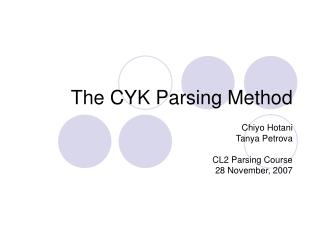Download PresentationThe CYK Parsing Method

# The CYK Parsing Method - PowerPoint PPT PresentationDownload Presentation## The CYK Parsing Method

- - - - - - - - - - - - - - - - - - - - - - - - - - - E N D - - - - - - - - - - - - - - - - - - - - - - - - - - -
##### Presentation Transcript

1. The CYK Parsing Method Chiyo Hotani Tanya Petrova CL2 Parsing Course 28 November, 2007

2. Overview • CYK Recognition with CF grammar • Basic Algorithm • Problems: unit-rules, є-rules • Recognition with a grammar in CNF • CYK Parsing with CNF • Parsing with CNF • Recognition Table • Chart Parsing • Summary • Advantages and Disadvantages • Other remarks

3. Basic Algorithm of CYK Recognition (1) Example Grammar: A grammar describing numbers in scientific notation Input: 32.5e+1

4. Basic Algorithm of CYK Recognition (2) Digit -> 0 | 1 | 2 | 3 | 4 | 5 | 6 | 7 | 8 | 9 Sign -> + | - derivations of substrings of length 1

5. Basic Algorithm of CYK Recognition (3) NumberS -> Integer | Real Integer -> Digit | Integer Digit Digit -> 0 | 1 | 2 | 3 | 4 | 5 | 6 | 7 | 8 | 9 derivations of substrings of length 1 • Unit Rule: rules of the form AB, where A and B are non-terminals. We can have chains of them in a derivation.

6. Basic Algorithm of CYK Recognition (4) NumberS -> Integer | Real Integer -> Digit | Integer Digit Fraction -> . Integer Scale -> e Sign Integer | Empty

7. Basic Algorithm of CYK Recognition (5) NumberS -> Integer | Real Real -> Integer Fraction Scale Number does indeed derive 32.5e+1.

8. Basic Algorithm of CYK Recognition (7) • Rє = { Empty, Scale } • sentence: z = z1z2 . . . znsubstring of z starting at position i, of length l.si,l = zizi+1. . . zi+l-1 • Rsi,l: the set of non-terminals deriving the substring si,l A graphical presentation of substrings

9. CYK recognition with a grammar in CNF • Required restrictions: • Eliminate є-rules and unit rules • Limit the maximum length of RHS of the rule to 2 • CNF • No є-rules and unit rules • all rules have one of the following two forms: AaABC

10. Our example grammar in CNF

11. CYK Parsing with CNF • Building the recognition table • Input : Our example grammar in CNF input sentence: 32.5 e + 1

12. CYK Parsing with the CNF • bottom-row : read directly from the grammar (rules of the form A a )

13. Two Ways to Copmute a R s i,l: • check each right-hand side • compute possible right-hand sides from the recognition table

14. How this is done Example: 2.5 e ( = s 2, 4) 1) N1 not in R s 2, 1 or R s 2, 2 N1 is a member of R s 2, 3 But Scale´ is not a member of R s 5, 1 2) R s 2, 4 is the set of Non- Terminals that have a right-hand side AB where either: A in R s 2, 1 and B in R s 3, 3 A in R s 2, 2 and B in R s 4, 2 A in R s 2, 3 and B in R s 5, 1 Possible combinations: N1 T2 or Number T2 In our grammar we do not have such a right-hand side, so nothing is added to R s 2, 4.

15. As a result we find out that: • This process is much less complicated than the one we saw before

16. Reasons • We do not have to repeat the process again and again until no new Non-Terminals are added to R s i,l (The substrings we are dealing with are really substrings and cannot be equal to the string we start with) • We only have to find one place where the substring must be split into two A  B C Here !

17. Chart Parsing A chart is just a recognition table.

18. A short retrospective of CYK • First: recognition table using the original grammar. • Then: transforming grammar to CNF.

19. A short retrospective of CYK cont. • CNF is useful for improving the efficiency, but it is actually a bit too restrictive  • Disadvantage of CNF: • Resulting recognition table lacks the information we need to construct a derivation using the original grammar!

20. A short retrospective of CYK cont. • In the transformation process, some non-terminals were thrown away (non-productive) • Missing information could be added.

21. A short retrospective of CYK cont. • Result: almost the same recognition table. • Extra information on non-terminals • Obtained in a simpler and much more efficient way.

22. Thank you for your attention! 